##### Statistical Analysis with Excel For Dummies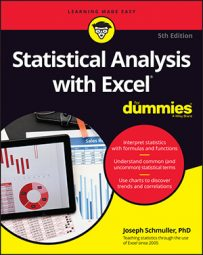Someday, Excel’s Analysis ToolPak might have a choice labeled ANOVA: Mixed Design. That day, unfortunately, is not today. Instead, you can use two ToolPak tools and knowledge about this type of design to provide the analysis.

The levels of the Between Group variable, Media (the A variable), are in the left column. The levels of the Within Group variable, Font (the B variable), are in the top row. Each cell entry is a reading speed in words per minute.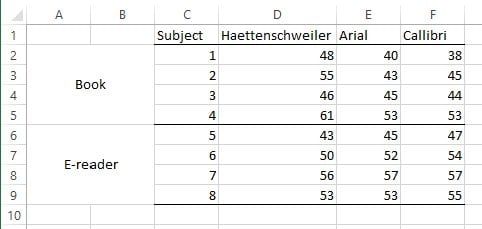Data for a study with a Between Group variable and a Within Group variable.

Next, you see the completed ANOVA table.

How do you get there? Surprisingly, it’s pretty easy, although quite a few steps are involved. All you have to do is run two ANOVAs on the same data and combine the ANOVA tables.

1. With the data entered into a worksheet, select Data | Data Analysis. This opens the Data Analysis dialog box.
2. From the Data Analysis dialog box, select Anova: Two-Factor Without Replication. This opens the Anova: Two-Factor Without Replication dialog box.
3. In the Input Range box, enter the cell range that holds the data. For this example, that’s C1:F9. This range includes the column headers, so select the Labels check box.
4. With the New Worksheet Ply radio button selected, click OK. The result is the ANOVA table.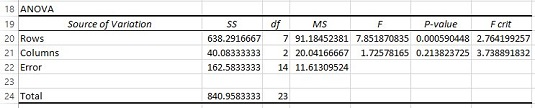5. Modify the ANOVA table: Insert rows for terms from the second ANOVA, change names of the Sources of Variance, and delete unnecessary values. First, insert four rows between Rows and Columns (between Row 20 and the original Row 21). Next, change Rows to Between Group and Columns to Font (the name of the B variable). Then, delete all the information from the row that has Error in the Source column. Finally, delete the F-ratios, P-values, and F-crits. The ANOVA table now looks like this.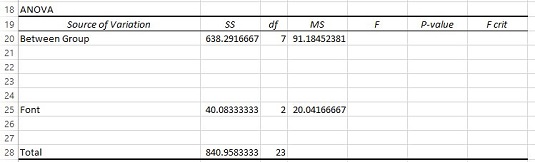6. Once again, select Data | Data Analysis.
7. This time, from the Data Analysis dialog box choose ANOVA: Two-Factor With Replication.
8. In the Input Range box, enter the cell array that holds the data, including the column headers. To do this, select C1:F9 in the worksheet.
9. In the Rows Per Sample box, enter the number of subjects within each level of the Between Groups variable. For this example, that’s 4.
10. With the New Worksheet Ply radio button selected, click OK.
11. Copy the resulting ANOVA table and paste it into the worksheet with the first ANOVA, just below the first ANOVA table. The worksheet should look like this.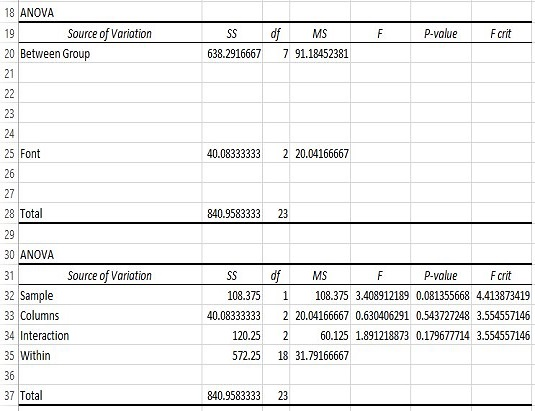12. Add Within Group to the first ANOVA table, four rows under Between Group, and calculate values for SS and df. Type Within Group into row 24. The SS for Within Group is the SS Total – SS Between Group (B28-B20). The df for Within Group is the df Total – df Between Group (C28-C20).
13. Copy the Sample row of data from the second ANOVA table and paste it into the first ANOVA table just below Between Group. Copy and paste just the Source name (Sample), its SS and its df.
14. Change Sample to the name of the Between Group variable (the A variable). Change Sample to Media.
15. In the next row, enter the name of the source for S/A, and calculate its SS, df, and MS. Enter Subject/Media. The SS is the SS Between Group – SS Media (B20:B21). The df is the df Between Group – df Media (C20:C21). The MS is the SS divided by the df (B22/C22).
16. In the appropriate cell, calculate the F ratio for the A variable. That’s MS Media divided by MS Subject/Media (D21/D22) calculated in E21. The ANOVA table now looks like this.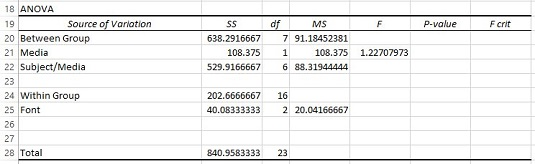17. From the second ANOVA table, copy the Interaction, its SS, its df, and its MS, and paste into the first ANOVA table in the row just below the name of the B variable. Change Interaction to the name of the interaction between the A variable and the B variable. Copy the information from Row 34 into Row 26, just below Font. Change Interaction to Media X Font.
18. In the next row, type the name of the source for B X S/A and calculate its SS, df, and MS. Type Font X Subject/Media into A27. The SS is the SS Within Group – SS Font – SS Media X Font (B24 – B25 – B26). The df is the df Within Group – df Font – df Media X Font (C24 – C25 – C26). The MS is the SS divided by the df (B27/C27).
19. In the appropriate cells, calculate the remaining F-ratios. In E25, divide D25 by D27. In E26, divide D26 by D27. For clarity, insert a row just above Total.
To make the table look like the completed ANOVA table shown earlier, use `F.DIST.RT` to find the P-values, and `F.INV.RT` to find the F-crits. You can also delete the value for MSBetween Group, because it serves no purpose.

For some nice cosmetic effects, indent the sources under the main categories (Between Groups and Within Groups), and center the df for the main categories.

And what about the analysis? The completed ANOVA table shows no effect of Media, a significant effect of Font, and a Media X Font interaction.

This procedure uses Anova: Two-Factor With Replication, a tool that depends on an equal number of replications (rows) for each combination of factors. So for this procedure to work, you have to have an equal number of people in each level of the Between Groups variable.